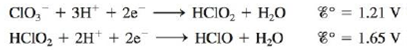Chapter 18, Problem 86E

Chapter
Section
Textbook Problem

# A disproportionation reaction involves a substance that acts as both an oxidizing and a reducing agent, producing higher and lower oxidation states of the same element in the products. Which of the following disproportionation reactions are spontaneous under standard conditions? Calculate ΔG° and K at 25°C for those reactions that are spontaneous under standard conditions.a. 2 Cu + ( a q ) → Cu 2 + ( a q ) + Cu ( s ) b. 3 Fe 2 + ( a q ) → 2 Fe 3 + ( a q ) + Fe ( s ) c. HClO 2 ( a q ) → ClO 3 − ( a q ) + HClO ( a q ) (unbalanced)Use the half-reactions:(a)

Interpretation Introduction

Interpretation:

The information regarding the disproportionation reaction is given. Various questions based on the spontaneity are to be answered and the calculation of ΔG° and K is to be stated.

Concept introduction:

The relationship between reduction potential and standard reduction potential value and activities of species present in an electrochemical cell at a given temperature is given by the Nernst equation.

The value of Ecell is calculated using Nernst formula,

E=E°(RTnF)ln(Q)

At room temperature the above equation is specified as,

E=E°(0.0591n)log(Q)

To determine: The spontaneity of the given reaction (a) and further calculation of ΔG° and K .

Explanation

Given

The disproportionation reaction is,

2Cu+(aq)Cu2+(aq)+Cu(s)

The reaction taking place at cathode is,

Cu+(aq)+eCu(s)E°red=0.52V

The reaction taking place at anode is,

Cu+(aq)Cu2+(aq)+eE°ox=0.16V

Add both the oxidation and reduction half-reaction,

Cu+(aq)+eCu(s)Cu+(aq)Cu2+(aq)+e

The final equation is,

2Cu+(aq)Cu2+(aq)+Cu(s)

The overall cell potential is calculated as,

E°cell=E°ox+E°red=0.16V+0.52V=0.36V

The relationship between cell potential and Gibbs free energy change is given by the formula,

ΔG°=nFE°cell

Where,

• ΔG° is the Gibbs free energy change at the standard conditions.
• n is the number of electrons involved in the reaction.
• F is the Faraday’s constant.
• E°cell is the cell potential at the standard condition.

As the value of E°cell comes out to be positive then ΔG° becomes negative. Therefore it leads to a spontaneous reaction.

The relationship between cell potential and Gibbs free energy change is given by the formula,

ΔG°=nFE°cell

The reaction involves the transfer of 1mole

Substitute the values of n , E°cell and F in the above equation as,

ΔG°=1×96,485×0

(b)

Interpretation Introduction

Interpretation:

The information regarding the disproportionation reaction is given. Various questions based on the spontaneity are to be answered and the calculation of ΔG° and K is to be stated.

Concept introduction:

The relationship between reduction potential and standard reduction potential value and activities of species present in an electrochemical cell at a given temperature is given by the Nernst equation.

The value of Ecell is calculated using Nernst formula,

E=E°(RTnF)ln(Q)

At room temperature the above equation is specified as,

E=E°(0.0591n)log(Q)

To determine: The spontaneity of the given reaction (b) and the value of ΔG° and K if the reaction is spontaneous.

(c)

Interpretation Introduction

Interpretation:

The information regarding the disproportionation reaction is given. Various questions based on the spontaneity are to be answered and the calculation of ΔG° and K is to be stated.

Concept introduction:

The relationship between reduction potential and standard reduction potential value and activities of species present in an electrochemical cell at a given temperature is given by the Nernst equation.

The value of Ecell is calculated using Nernst formula,

E=E°(RTnF)ln(Q)

At room temperature the above equation is specified as,

E=E°(0.0591n)log(Q)

To determine: The spontaneity of the given reaction (c) and further calculation of ΔG° and K if the reaction is spontaneous.

### Still sussing out bartleby?

Check out a sample textbook solution.

See a sample solution

#### The Solution to Your Study Problems

Bartleby provides explanations to thousands of textbook problems written by our experts, many with advanced degrees!

Get Started